# 机器学习系列(14)_SVM碎碎念part2：SVM中的向量与空间距离

## 2.从向量到距离计算

SVM = Support Vector Machine，我们在Support Vector Machine中, 看到这个单词-vector（向量）。是的，SVM中的大量计算都是建立在向量基础上的，所以这篇做一个简短的知识回顾，会涉及到的内容包括：

• 向量是什么
• 它的模长
• 它的方向
• 如何加减向量
• 什么是点积
• 如何将一个向量映射到另一个向量上
• 超平面的方程是什么
• 如何计算间隔

### 2.1 什么是向量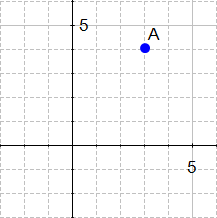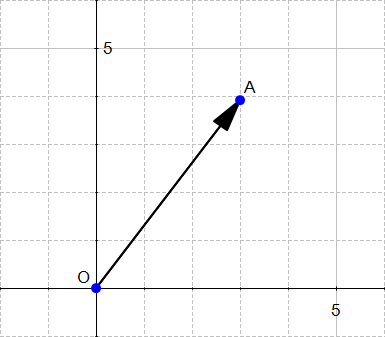OK，所以这里涉及到两个概念：大小方向

#### 1) 向量大小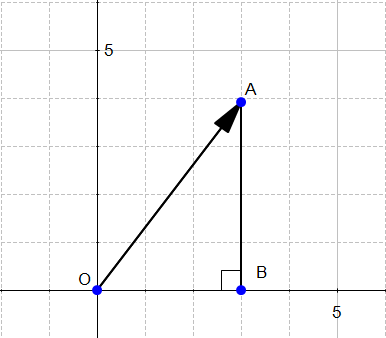OA2=OB2+AB2OA2=32+42OA2=25OA=25OA=OA=5

#### 2) 向量的方向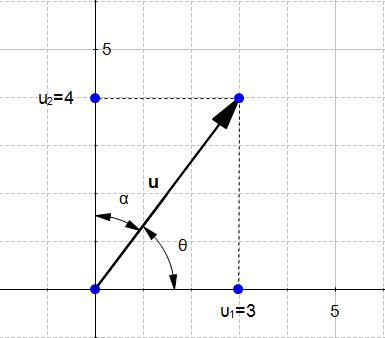cos(θ)=u1ucos(α)=u2u

#### 计算方向向量

cos(θ)=u1u=35=0.6cos(α)=u2u=45=0.8### 两个向量的和u+v=(u1+v1,u2+v2)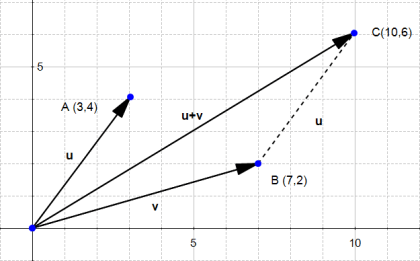### 两个向量的差

uv=(u1v1,u2v2)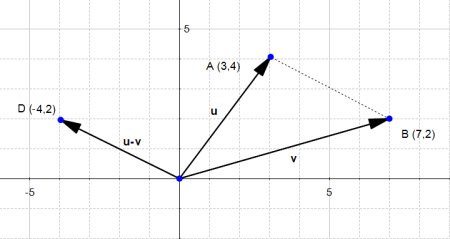vu=(v1u1,v2u2)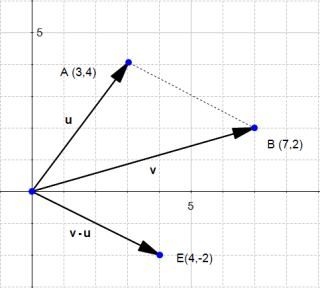xy=xycos(θ)

### 为什么内积这么算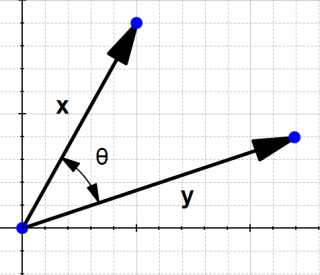cos(β)=

OK，一个稍微复杂一点的图形，里面有两个向量，如下：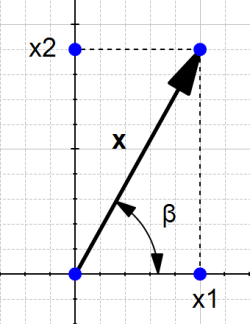θ=βα

cos(βα)=cos(β)cos(α)+sin(β)sin(α)

(忘记这部分高中数学知识的同学请点击公式推导)

cos(β)==x1xsin(β)==x2xcos(α)==y1ysin(α)==y2y

cos(θ)=cos(βα)=cos(β)cos(α)+sin(β)sin(α)cos(θ)=x1xy1y+x2xy2ycos(θ)=x1y1+x2y2xy

xy$\|x\|\|y\|$左移有：

xycos(θ)=x1y1+x2y2

xycos(θ)=xy

• 向量X，Y的内积（线性代数）
• 标量积，因为我们做两个向量的乘积，它返回一个标量（一个实数）。

### 向量的正交投影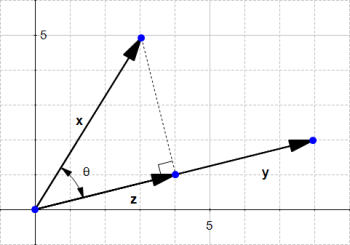cos(θ)=zxz=xcos(θ)

cos(θ)=xyxy

z=xxyxyz=xyy

u=yy

z=ux

z$\mathbf{z}$y$\mathbf{y}$有相同的方向向量u$\mathbf{u}$

u=zzz=zu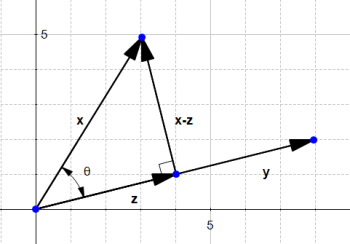xz=(34)2+(51)2=17

## 3. SVM的超平面

wTx=0

wTx=b×(1)+(a)×x+1×ywTx=yaxb

• 在多于二维的空间里，这个方程式更适用
• 向量w垂直于超平面

### 3.1 计算点到超平面距离wTx=0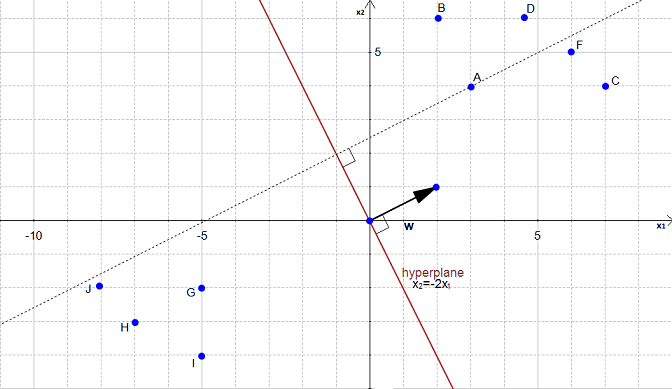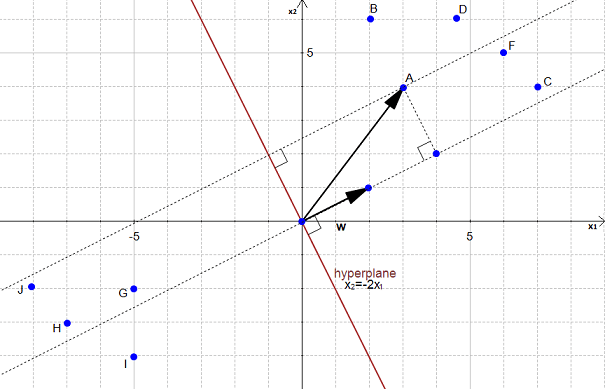w=22+12=5

u=(25,15)

p$\mathbf{p}$a$\mathbf{a}$w$\mathbf{w}$上的投影，所以：
p=(ua)up=(3×25+4×15)up=(65+45)up=105up=(105×25,105×15)p=(205,105)p=(4,2)p=42+22=25

margin=2p=45

## 4.总结

OK，到目前为止，其实就是简单回顾了一下向量中的一些概念，依旧用向量的知识，怎么帮助我们去计算超平面间隔，有兴趣的同学请接着看part3:如何找到最优分离超平面©️2019 CSDN 皮肤主题: 编程工作室 设计师: CSDN官方博客